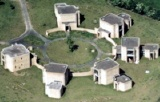# Mathematics Colloquia Abstracts

## Abstracts

Abstracts will be added as they become available.

Friday 27 April 2007

Charles Fefferman (Princeton)
Whitney's extension problems

Infinite flavor: Fix m,n>=1. Let E be a (completely arbitrary) subset of R^n, and let f:E->R be a function. How can we tell whether f extends to a C^m function on the whole R^n? If F exists, then about how small can we take its C^m norm? What can we say about the derivatives of F at a given point? Can we take F to depend linearly on f?

finite flavor: Fix m,n>=1, and let x1,...,xN be given points in R^n. How can we compute a function F on R^n, whose graph passes through (or close to) all (or all but a few) of the given points, with the C^m norm of F almost as small as possible?

The work on the finite flavor is joint work with Bo'az Klartag.

Friday 4 May 2007
John Ockendon (Oxford)
Some singularity problems in theoretical mechanics

This talk will comprise brief descriptions of the mathematical challenges posed by modelling
(i) dislocation pile-ups using singular solutions of the equations of elasticity;
(ii) supersonic shaped-charge penetration;
(iii) fast uphill shallow-water flow; and
(iv) sonic boom.

Friday 11 May 2007
Gareth Jones (Southampton)
Complete bipartite maps, factorisations of groups, and generalised Fermat curves

Grothendieck's theory of dessins d'enfants uses maps (i.e. embedded graphs) on surfaces to link the Teichmueller theory of compact Riemann surfaces with the Galois theory of algebraic number fields and the projective algebraic curves defined over them. For instance, the Fermat curve \$x^n + y^n + z^n = 0\$, of genus \$(n-1)(n-2)/2\$, corresponds to the so-called standard embedding of the complete bipartite graph \$K_{n,n}\$. Using results of Huppert, Ito and Wielandt on factorisations of finite groups, joint work with Du, Kwak, Nedela and Skoviera has classified the regular (i.e. arc-transitive) embeddings of \$K_{n,n}\$ when \$n\$ is a prime power, and joint work with Coste, Streit and Wolfart has determined the fields of definition, the Galois orbits, and in some cases explicit equations for the corresponding generalised Fermat curves. Using Hall's theorems on finite solvable groups, this classification of embeddings has recently been extended to all \$n\$, and work is now beginning on the corresponding Galois theory.

Friday 18 May 2007
Geoffrey Grimmett (Cambridge)
Classical and quantum ferromagnets via stochastic geometry

We describe how stochastic geometry may be used to study the Ising model for the ferromagnet. The two main techniques are the random-cluster and the random-current methods, and the first of these may be used also to derive properties of the quantum Ising model.

Friday 25 May 2007
Soap bubbles, integrable systems and singular pertubation theory

The mathematical idealization of static soap films and soap bubbles are minimal surfaces and constant mean curvature (CMC) surfaces respectively, and have been studied by mathematicians since at least the 19th century. In the 1950s two fundamental results by Hopf and Alexandrov proved that under some conditions 'soap bubbles must be round' and led to a conjecture that this was always the case (the Hopf conjecture).

In the mid 1980s Wente disproved the Hopf conjecture by constructing 'soap bubbles' which are tori. Subsequently various mathematicians realized that all CMC tori could be understood using methods adapted from mathematical physics, namely (infinitely-dimensional) completely integrable Hamiltonian systems.

Then in the early 1990s Kapouleas constructed many compact CMC surfaces of higher genus using a geometric/analytic method inspired by singular perturbation theory.

This talk will try to explain the basic features of CMC surfaces, how integrable systems and singular perturbation theory arise in this geometric context and how they can can be used to construct compact 'soap bubbles'. We hope the talk will be accessible to a general maths audience.

Friday 1 June 2007
John Hubbard (Cornell and Marseille)
The Weil Conjectures and Etale Topology

Friday 8 June 2007
Andrew Stuart (Warwick)
Markov Chain Monte Carlo methods in infinite dimensions

Friday 15 June 2007
Alastair King (Bath)
Why are moduli spaces projective varieties?

Iwill explain how certain moduli spaces have natural homogeneous coordinates, illustrating the point with three examples: Plucker coordinates on Grassmannians, determinantal semi-invariants of Kronecker modules and theta functions on moduli of coherent sheaves on projective varieties.Internet Access at Warwick:
Where possible, visitors should obtain an EDUROAM account from their own university to enable internet access whilst at Warwick.
If you need WiFi whilst at Warwick, click here for instructions (upon arrival at Warwick)
Registration:
You can register for any of the symposia or workshops online. To see which registrations are currently open and to submit a registration, please click here.
Contact:
Mathematics Research Centre
Zeeman Building
University of Warwick
Coventry CV4 7AL - UK
E-mail:
mrc@maths.warwick.ac.uk